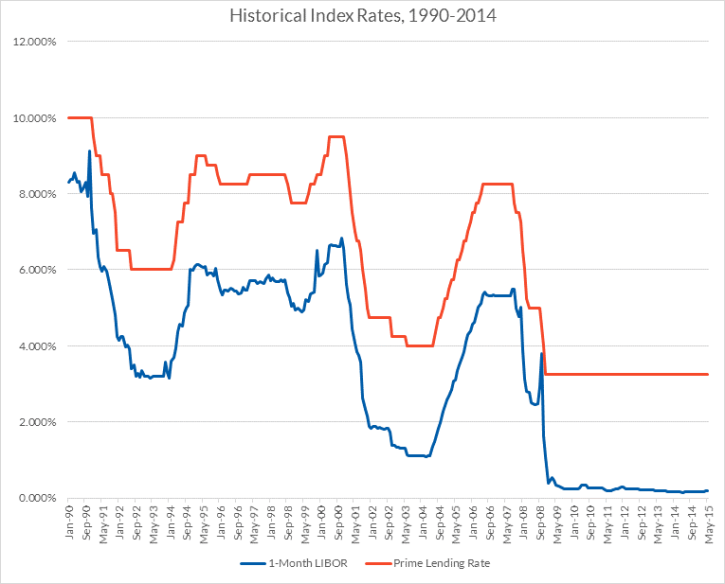Home College Loans Understanding Student Loan Terminology Fixed vs. Variable Interest Rates

# Fixed vs. Variable Interest Rates

Borrowers are sometimes faced with a choice between a fixed-rate and a variable-rate loan. Which type of interest rate is better often depends on the borrower’s circumstances and preferences.

Find & Compare Private Student Loans for Your School:

CONTENTS:

## Definition of Fixed and Variable Interest Rates

Fixed interest rates do not change over the life of the loan.

Variable interest rates (sometimes called floating rates) may change periodically. The interest rate may reset on a monthly, quarterly or annual basis, depending on the terms of the loan.

Variable interest rates are expressed as the sum of an index rate, which changes periodically, and a fixed margin. Examples of index rates include the high yield on the 10-year Treasury Note, the 91-day T-Bill rate, the 1-month and 3-month average London Interbank Offered Rate (LIBOR) and the Prime Lending Rate.

Don’t ignore the index on a variable-rate loan. A variable-rate loan with interest rate equal to 1M LIBOR + 3% might start off at 3.25% when the 1-month LIBOR is 0.25%, but could rise to 7.5% if the 1-month LIBOR increases to 4.5%. Borrowers sometimes incorrectly assume that a 1M LIBOR + 3% variable-rate loan is a 3% fixed-rate loan. Or, they may base loan calculations on the initial 3.25% interest rate without considering the possibility of a much higher interest rate later. This can cause borrowers to underestimate the cost of a variable-rate loan.

## Impact of Fixed and Variable Interest Rates

A fixed interest rate provides stability and predictability. The monthly payment on a fixed-rate loan will remain unchanged, assuming a level amortization repayment plan, where all of the monthly payments are the same.

The loan payments on a variable-rate loan, on the other hand, may change periodically, sometimes as frequently as monthly. This may make it harder for the borrower to predict and budget for changes in the monthly payment.

When interest rates are expected to rise, a variable interest rate will generally start off lower than the equivalent fixed interest rate. The variable interest rate may increase as prevailing interest rates rise, eventually exceeding the fixed interest rate. If interest rates rise faster than expected, the variable-rate loan may cost more over the life of the loan than the fixed-rate loan. If interest rates rise slower than expected, or even decrease, the variable-rate loan may cost less over the life of the loan.

Every percentage point increase in the interest rate on a variable-rate loan will increase the monthly payment by about 4.5% to 5% on a 10-year repayment term, 8% to 10% on a 20-year term and 10% to 15% on a 30-year term.

The length of the loan term can affect the pricing of a fixed interest rate. In a rising interest rate environment, lenders offering loans with fixed interest rates may restrict the loans to shorter repayment terms. This limits the lender’s exposure to a higher cost of funds as prevailing interest rates rise. So, generally, a lower fixed interest rate will mean a shorter repayment term.

For example, if interest rates are expected to increase by 1.5 percentage points per year over a four-year period, followed by six years of stable interest rates, a lender might offer a fixed interest rate that is 4.0 percentage points higher than the initial variable interest rate, assuming a 10-year repayment term. With a 5-year repayment term, the fixed interest rate is 3.1 percentage points higher than the initial variable interest rate, and with a 3-year term the difference in interest rates drops to 2.7 percentage points.

When comparing two fixed-rate loans, it is best to limit the comparison to loans that have the same repayment term. Otherwise, the difference in the interest rate may have more to do with the differences in the repayment terms than in the underlying cost of the loan. For example, while the annual percentage rate (APR) rolls fees into the interest rate to make loans more comparable, it is not a useful tool for comparing loans with different repayment terms. If two loans differ only in the repayment terms, the loan with the longer repayment term will have the lower APR as it amortizes the fees over a longer period of time, even though this loan will cost the borrower more over the life of the loan.

With a variable-rate loan, on the other hand, the lender may have more flexibility to offer loans with longer repayment terms, since the spread between the borrower’s interest rate and the lender’s cost of funds may be constant, despite changes in the prevailing interest rates.

Whether a borrower prefers a fixed interest rate or a variable interest rate may depend on whether interest rates are expected to increase or decrease and the borrower’s risk tolerance.  Borrowers who are in repayment longer may be less willing to risk an increase in the cost of their loans.

Borrowers who are planning on paying off their loans early may be better off with a variable-rate loan, especially if they are able to pay off the debt before interest rates rise too much.

Currently, interest rates are at historic lows, so interest rates are more likely to go up than to go down. In a way, variable interest rates have nowhere to go but up.